# Python Data Structures

• Difficulty Level : Easy
• Last Updated : 10 May, 2022

Data Structures are a way of organizing data so that it can be accessed more efficiently depending upon the situation. Data Structures are fundamentals of any programming language around which a program is built. Python helps to learn the fundamental of these data structures in a simpler way as compared to other programming languages.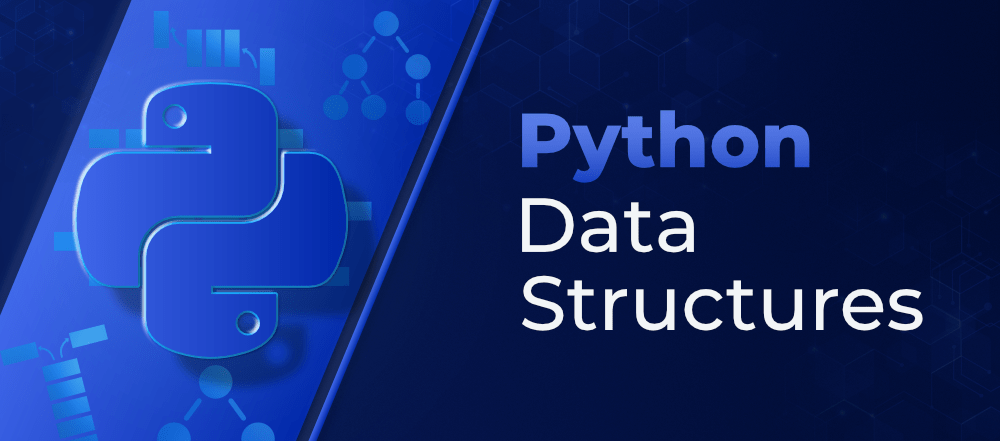In this article, we will discuss the Data Structures in the Python Programming Language and how they are related to some specific Python Data Types. We will discuss all the in-built data structures like list tuples, dictionaries, etc. as well as some advanced data structures like trees, graphs, etc.## Lists

Python Lists are just like the arrays, declared in other languages which is an ordered collection of data. It is very flexible as the items in a list do not need to be of the same type.

The implementation of Python List is similar to Vectors in C++ or ArrayList in JAVA. The costly operation is inserting or deleting the element from the beginning of the List as all the elements are needed to be shifted. Insertion and deletion at the end of the list can also become costly in the case where the preallocated memory becomes full.

We can create a list in python as shown below.

## Python3

 `List` `=` `[``1``, ``2``,  ``3``, ``"GFG"``, ``2.3``]``print``(``List``)`
Output
`[1, 2, 3, 'GFG', 2.3]`

List elements can be accessed by the assigned index. In python starting index of the list, sequence is 0 and the ending index is (if N elements are there) N-1.## Python3

 `# Creating a List with``# the use of multiple values``List` `=` `[``"Geeks"``, ``"For"``, ``"Geeks"``]``print``(``"\nList containing multiple values: "``)``print``(``List``)` `# Creating a Multi-Dimensional List``# (By Nesting a list inside a List)``List2 ``=` `[[``'Geeks'``, ``'For'``], [``'Geeks'``]]``print``(``"\nMulti-Dimensional List: "``)``print``(List2)` `# accessing a element from the ``# list using index number``print``(``"Accessing element from the list"``)``print``(``List``[``0``]) ``print``(``List``[``2``])` `# accessing a element using``# negative indexing``print``(``"Accessing element using negative indexing"``)``    ` `# print the last element of list``print``(``List``[``-``1``])``    ` `# print the third last element of list ``print``(``List``[``-``3``])`
Output
```List containing multiple values:
['Geeks', 'For', 'Geeks']

Multi-Dimensional List:
[['Geeks', 'For'], ['Geeks']]
Accessing element from the list
Geeks
Geeks
Accessing element using negative indexing
Geeks
Geeks```

## Dictionary

Python dictionary is like hash tables in any other language with the time complexity of O(1). It is an unordered collection of data values, used to store data values like a map, which, unlike other Data Types that hold only a single value as an element, Dictionary holds the key:value pair. Key-value is provided in the dictionary to make it more optimized.

Indexing of Python Dictionary is done with the help of keys. These are of any hashable type i.e. an object whose can never change like strings, numbers, tuples, etc. We can create a dictionary by using curly braces ({}) or dictionary comprehension.

## Python3

 `# Creating a Dictionary``Dict` `=` `{``'Name'``: ``'Geeks'``, ``1``: [``1``, ``2``, ``3``, ``4``]}``print``(``"Creating Dictionary: "``)``print``(``Dict``)` `# accessing a element using key``print``(``"Accessing a element using key:"``)``print``(``Dict``[``'Name'``])` `# accessing a element using get()``# method``print``(``"Accessing a element using get:"``)``print``(``Dict``.get(``1``))` `# creation using Dictionary comprehension``myDict ``=` `{x: x``*``*``2` `for` `x ``in` `[``1``,``2``,``3``,``4``,``5``]}``print``(myDict)`
Output
```Creating Dictionary:
{'Name': 'Geeks', 1: [1, 2, 3, 4]}
Accessing a element using key:
Geeks
Accessing a element using get:
[1, 2, 3, 4]
{1: 1, 2: 4, 3: 9, 4: 16, 5: 25}```

## Tuple

Python Tuple is a collection of Python objects much like a list but Tuples are immutable in nature i.e. the elements in the tuple cannot be added or removed once created. Just like a List, a Tuple can also contain elements of various types.

In Python, tuples are created by placing a sequence of values separated by ‘comma’ with or without the use of parentheses for grouping of the data sequence.

Note: Tuples can also be created with a single element, but it is a bit tricky. Having one element in the parentheses is not sufficient, there must be a trailing ‘comma’ to make it a tuple.

## Python3

 `# Creating a Tuple with``# the use of Strings``Tuple` `=` `(``'Geeks'``, ``'For'``)``print``(``"\nTuple with the use of String: "``)``print``(``Tuple``)``    ` `# Creating a Tuple with``# the use of list``list1 ``=` `[``1``, ``2``, ``4``, ``5``, ``6``]``print``(``"\nTuple using List: "``)``Tuple` `=` `tuple``(list1)` `# Accessing element using indexing``print``(``"First element of tuple"``)``print``(``Tuple``[``0``])` `# Accessing element from last``# negative indexing``print``(``"\nLast element of tuple"``)``print``(``Tuple``[``-``1``])``  ` `print``(``"\nThird last element of tuple"``)``print``(``Tuple``[``-``3``])`
Output
```Tuple with the use of String:
('Geeks', 'For')

Tuple using List:
First element of tuple
1

Last element of tuple
6

Third last element of tuple
4```

## Set

Python Set is an ordered collection of data that is mutable and does not allow any duplicate element. Sets are basically used to include membership testing and eliminating duplicate entries. The data structure used in this is Hashing, a popular technique to perform insertion, deletion, and traversal in O(1) on average.

If Multiple values are present at the same index position, then the value is appended to that index position, to form a Linked List. In, CPython Sets are implemented using a dictionary with dummy variables, where key beings the members set with greater optimizations to the time complexity.

Set Implementation: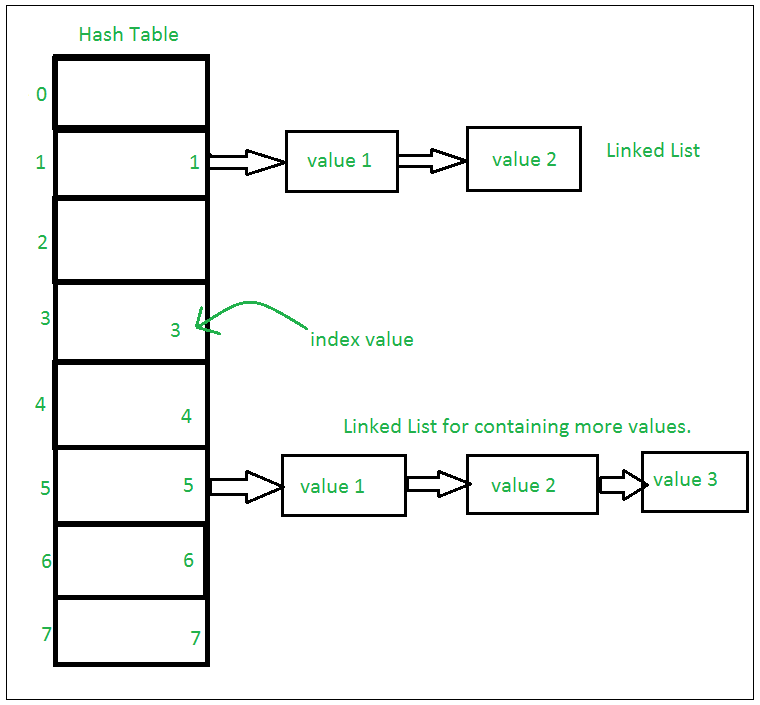Sets with Numerous operations on a single HashTable: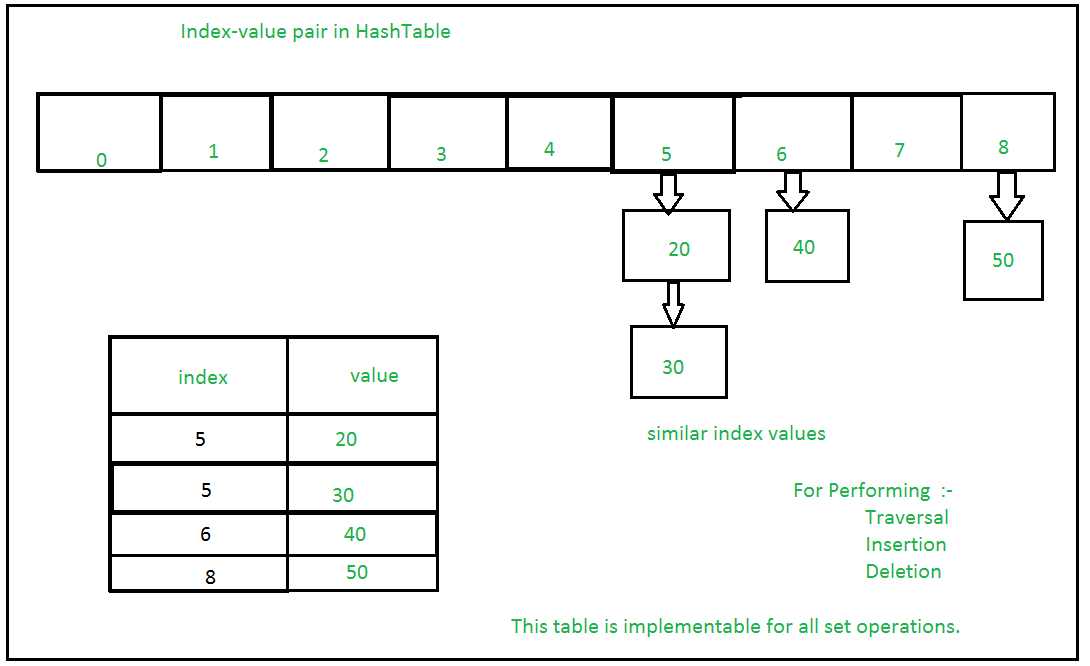## Python3

 `# Creating a Set with ``# a mixed type of values``# (Having numbers and strings)``Set` `=` `set``([``1``, ``2``, ``'Geeks'``, ``4``, ``'For'``, ``6``, ``'Geeks'``])``print``(``"\nSet with the use of Mixed Values"``)``print``(``Set``)` `# Accessing element using``# for loop``print``(``"\nElements of set: "``)``for` `i ``in` `Set``:``    ``print``(i, end ``=``" "``)``print``()` `# Checking the element``# using in keyword``print``(``"Geeks"` `in` `Set``)`
Output
```Set with the use of Mixed Values
{1, 2, 'Geeks', 4, 6, 'For'}

Elements of set:
1 2 Geeks 4 6 For
True```

## Frozen Sets

Frozen sets in Python are immutable objects that only support methods and operators that produce a result without affecting the frozen set or sets to which they are applied. While elements of a set can be modified at any time, elements of the frozen set remain the same after creation.

If no parameters are passed, it returns an empty frozenset.

## Python3

 `# Same as {"a", "b","c"}``normal_set ``=` `set``([``"a"``, ``"b"``,``"c"``])` `print``(``"Normal Set"``)``print``(normal_set)` `# A frozen set``frozen_set ``=` `frozenset``([``"e"``, ``"f"``, ``"g"``])` `print``(``"\nFrozen Set"``)``print``(frozen_set)` `# Uncommenting below line would cause error as``# we are trying to add element to a frozen set``# frozen_set.add("h")`
Output
```Normal Set
{'a', 'c', 'b'}

Frozen Set
frozenset({'g', 'e', 'f'})```

## String

Python Strings are arrays of bytes representing Unicode characters. In simpler terms, a string is an immutable array of characters. Python does not have a character data type, a single character is simply a string with a length of 1.

Note: As strings are immutable, modifying a string will result in creating a new copy.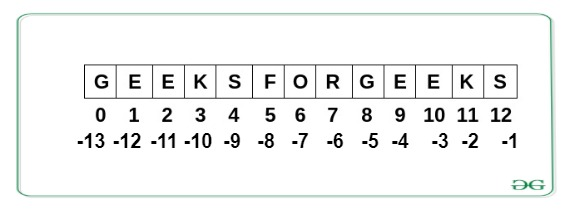## Python3

 `String ``=` `"Welcome to GeeksForGeeks"``print``(``"Creating String: "``)``print``(String)``    ` `# Printing First character``print``(``"\nFirst character of String is: "``)``print``(String[``0``])``    ` `# Printing Last character``print``(``"\nLast character of String is: "``)``print``(String[``-``1``])`
Output
```Creating String:
Welcome to GeeksForGeeks

First character of String is:
W

Last character of String is:
s```

## Bytearray

Python Bytearray gives a mutable sequence of integers in the range 0 <= x < 256.

## Python3

 `# Creating bytearray``a ``=` `bytearray((``12``, ``8``, ``25``, ``2``))``print``(``"Creating Bytearray:"``)``print``(a)` `# accessing elements``print``(``"\nAccessing Elements:"``, a[``1``])` `# modifying elements``a[``1``] ``=` `3``print``(``"\nAfter Modifying:"``)``print``(a)` `# Appending elements``a.append(``30``)``print``(``"\nAfter Adding Elements:"``)``print``(a)`
Output
```Creating Bytearray:
bytearray(b'\x0c\x08\x19\x02')

Accessing Elements: 8

After Modifying:
bytearray(b'\x0c\x03\x19\x02')

bytearray(b'\x0c\x03\x19\x02\x1e')```

Till now we have studied all the data structures that come built-in into core Python. Now let dive more deep into Python and see the collections module that provides some containers that are useful in many cases and provide more features than the above-defined functions.

## Collections Module

Python collection module was introduced to improve the functionality of the built-in datatypes. It provides various containers let’s see each one of them in detail.

### Counters

A counter is a sub-class of the dictionary. It is used to keep the count of the elements in an iterable in the form of an unordered dictionary where the key represents the element in the iterable and value represents the count of that element in the iterable. This is equivalent to a bag or multiset of other languages.

## Python3

 `from` `collections ``import` `Counter``  ` `# With sequence of items``print``(Counter([``'B'``,``'B'``,``'A'``,``'B'``,``'C'``,``'A'``,``'B'``,``'B'``,``'A'``,``'C'``]))``  ` `# with dictionary``count ``=` `Counter({``'A'``:``3``, ``'B'``:``5``, ``'C'``:``2``})``print``(count)` `count.update([``'A'``, ``1``])``print``(count)`
Output
```Counter({'B': 5, 'A': 3, 'C': 2})
Counter({'B': 5, 'A': 3, 'C': 2})
Counter({'B': 5, 'A': 4, 'C': 2, 1: 1})```

### OrderedDict

An OrderedDict is also a sub-class of dictionary but unlike a dictionary, it remembers the order in which the keys were inserted.

## Python3

 `from` `collections ``import` `OrderedDict` `print``(``"Before deleting:\n"``)``od ``=` `OrderedDict()``od[``'a'``] ``=` `1``od[``'b'``] ``=` `2``od[``'c'``] ``=` `3``od[``'d'``] ``=` `4` `for` `key, value ``in` `od.items():``    ``print``(key, value)` `print``(``"\nAfter deleting:\n"``)``od.pop(``'c'``)``for` `key, value ``in` `od.items():``    ``print``(key, value)` `print``(``"\nAfter re-inserting:\n"``)``od[``'c'``] ``=` `3``for` `key, value ``in` `od.items():``    ``print``(key, value)`
Output
```Before deleting:

a 1
b 2
c 3
d 4

After deleting:

a 1
b 2
d 4

After re-inserting:

a 1
b 2
d 4
c 3```

### DefaultDict

DefaultDict is used to provide some default values for the key that does not exist and never raises a KeyError. Its objects can be initialized using DefaultDict() method by passing the data type as an argument.

Note: default_factory is a function that provides the default value for the dictionary created. If this parameter is absent then the KeyError is raised.

## Python3

 `from` `collections ``import` `defaultdict``    `  `# Defining the dict``d ``=` `defaultdict(``int``)``    ` `L ``=` `[``1``, ``2``, ``3``, ``4``, ``2``, ``4``, ``1``, ``2``]``    ` `# Iterate through the list``# for keeping the count``for` `i ``in` `L:``        ` `    ``# The default value is 0``    ``# so there is no need to``    ``# enter the key first``    ``d[i] ``+``=` `1``        ` `print``(d)`
Output
`defaultdict(<class 'int'>, {1: 2, 2: 3, 3: 1, 4: 2})`

### ChainMap

A ChainMap encapsulates many dictionaries into a single unit and returns a list of dictionaries. When a key is needed to be found then all the dictionaries are searched one by one until the key is found.

## Python3

 `from` `collections ``import` `ChainMap``    ` `    ` `d1 ``=` `{``'a'``: ``1``, ``'b'``: ``2``}``d2 ``=` `{``'c'``: ``3``, ``'d'``: ``4``}``d3 ``=` `{``'e'``: ``5``, ``'f'``: ``6``}``    ` `# Defining the chainmap``c ``=` `ChainMap(d1, d2, d3)``print``(c)` `print``(c[``'a'``])``print``(c[``'g'``])`

Output

```ChainMap({'a': 1, 'b': 2}, {'c': 3, 'd': 4}, {'e': 5, 'f': 6})
1```
`KeyError: 'g'`

### NamedTuple

A NamedTuple returns a tuple object with names for each position which the ordinary tuples lack. For example, consider a tuple names student where the first element represents fname, second represents lname and the third element represents the DOB. Suppose for calling fname instead of remembering the index position you can actually call the element by using the fname argument, then it will be really easy for accessing tuples element. This functionality is provided by the NamedTuple.

## Python3

 `from` `collections ``import` `namedtuple``    ` `# Declaring namedtuple()``Student ``=` `namedtuple(``'Student'``,[``'name'``,``'age'``,``'DOB'``])``    ` `# Adding values``S ``=` `Student(``'Nandini'``,``'19'``,``'2541997'``)``    ` `# Access using index``print` `(``"The Student age using index is : "``,end ``=``"")``print` `(S[``1``])``    ` `# Access using name``print` `(``"The Student name using keyname is : "``,end ``=``"")``print` `(S.name)`
Output
```The Student age using index is : 19
The Student name using keyname is : Nandini```

### Deque

Deque (Doubly Ended Queue) is the optimized list for quicker append and pop operations from both sides of the container. It provides O(1) time complexity for append and pop operations as compared to the list with O(n) time complexity.

Python Deque is implemented using doubly linked lists therefore the performance for randomly accessing the elements is O(n).

## Python3

 `# importing "collections" for deque operations``import` `collections` `# initializing deque``de ``=` `collections.deque([``1``,``2``,``3``])` `# using append() to insert element at right end``# inserts 4 at the end of deque``de.append(``4``)` `# printing modified deque``print``(``"The deque after appending at right is : "``)``print``(de)` `# using appendleft() to insert element at left end``# inserts 6 at the beginning of deque``de.appendleft(``6``)` `# printing modified deque``print``(``"The deque after appending at left is : "``)``print``(de)` `# using pop() to delete element from right end``# deletes 4 from the right end of deque``de.pop()` `# printing modified deque``print``(``"The deque after deleting from right is : "``)``print``(de)` `# using popleft() to delete element from left end``# deletes 6 from the left end of deque``de.popleft()` `# printing modified deque``print``(``"The deque after deleting from left is : "``)``print``(de)`
Output
```The deque after appending at right is :
deque([1, 2, 3, 4])
The deque after appending at left is :
deque([6, 1, 2, 3, 4])
The deque after deleting from right is :
deque([6, 1, 2, 3])
The deque after deleting from left is :
deque([1, 2, 3])```

## UserDict

UserDict is a dictionary-like container that acts as a wrapper around the dictionary objects. This container is used when someone wants to create their own dictionary with some modified or new functionality.

## Python3

 `from` `collections ``import` `UserDict` `# Creating a Dictionary where``# deletion is not allowed``class` `MyDict(UserDict):``    ` `    ``# Function to stop deletion``    ``# from dictionary``    ``def` `__del__(``self``):``        ``raise` `RuntimeError(``"Deletion not allowed"``)``        ` `    ``# Function to stop pop from``    ``# dictionary``    ``def` `pop(``self``, s ``=` `None``):``        ``raise` `RuntimeError(``"Deletion not allowed"``)``        ` `    ``# Function to stop popitem``    ``# from Dictionary``    ``def` `popitem(``self``, s ``=` `None``):``        ``raise` `RuntimeError(``"Deletion not allowed"``)``    ` `# Driver's code``d ``=` `MyDict({``'a'``:``1``,``    ``'b'``: ``2``,``    ``'c'``: ``3``})` `print``(``"Original Dictionary"``)``print``(d)` `d.pop(``1``)`

Output

```Original Dictionary
{'a': 1, 'b': 2, 'c': 3}```
`RuntimeError: Deletion not allowed`

### UserList

UserList is a list-like container that acts as a wrapper around the list objects. This is useful when someone wants to create their own list with some modified or additional functionality.

## Python3

 `# Python program to demonstrate``# userlist`  `from` `collections ``import` `UserList`  `# Creating a List where``# deletion is not allowed``class` `MyList(UserList):``    ` `    ``# Function to stop deletion``    ``# from List``    ``def` `remove(``self``, s ``=` `None``):``        ``raise` `RuntimeError(``"Deletion not allowed"``)``        ` `    ``# Function to stop pop from``    ``# List``    ``def` `pop(``self``, s ``=` `None``):``        ``raise` `RuntimeError(``"Deletion not allowed"``)``    ` `# Driver's code``L ``=` `MyList([``1``, ``2``, ``3``, ``4``])` `print``(``"Original List"``)``print``(L)` `# Inserting to List"``L.append(``5``)``print``(``"After Insertion"``)``print``(L)` `# Deleting From List``L.remove()`

Output

```Original List
[1, 2, 3, 4]
After Insertion
[1, 2, 3, 4, 5]```
`RuntimeError: Deletion not allowed`

### UserString

UserString is a string-like container and just like UserDict and UserList, it acts as a wrapper around string objects. It is used when someone wants to create their own strings with some modified or additional functionality.

## Python3

 `from` `collections ``import` `UserString` `# Creating a Mutable String``class` `Mystring(UserString):``    ` `    ``# Function to append to``    ``# string``    ``def` `append(``self``, s):``        ``self``.data ``+``=` `s``        ` `    ``# Function to remove from``    ``# string``    ``def` `remove(``self``, s):``        ``self``.data ``=` `self``.data.replace(s, "")``    ` `# Driver's code``s1 ``=` `Mystring(``"Geeks"``)``print``(``"Original String:"``, s1.data)` `# Appending to string``s1.append(``"s"``)``print``(``"String After Appending:"``, s1.data)` `# Removing from string``s1.remove(``"e"``)``print``(``"String after Removing:"``, s1.data)`
Output
```Original String: Geeks
String After Appending: Geekss
String after Removing: Gkss```

Now after studying all the data structures let’s see some advanced data structures such as stack, queue, graph, linked list, etc. that can be used in Python Language.

A linked list is a linear data structure, in which the elements are not stored at contiguous memory locations. The elements in a linked list are linked using pointers as shown in the below image:A linked list is represented by a pointer to the first node of the linked list. The first node is called the head. If the linked list is empty, then the value of the head is NULL. Each node in a list consists of at least two parts:

• Data
• Pointer (Or Reference) to the next node

## Python3

 `# Node class``class` `Node:` `    ``# Function to initialize the node object``    ``def` `__init__(``self``, data):``        ``self``.data ``=` `data ``# Assign data``        ``self``.``next` `=` `None` `# Initialize``                        ``# next as null` `# Linked List class``class` `LinkedList:``    ` `    ``# Function to initialize the Linked``    ``# List object``    ``def` `__init__(``self``):``        ``self``.head ``=` `None`

Let us create a simple linked list with 3 nodes.

## Python3

 `# A simple Python program to introduce a linked list` `# Node class``class` `Node:` `    ``# Function to initialise the node object``    ``def` `__init__(``self``, data):``        ``self``.data ``=` `data ``# Assign data``        ``self``.``next` `=` `None` `# Initialize next as null`  `# Linked List class contains a Node object``class` `LinkedList:` `    ``# Function to initialize head``    ``def` `__init__(``self``):``        ``self``.head ``=` `None`  `# Code execution starts here``if` `__name__``=``=``'__main__'``:` `    ``# Start with the empty list``    ``llist ``=` `LinkedList()` `    ``llist.head ``=` `Node(``1``)``    ``second ``=` `Node(``2``)``    ``third ``=` `Node(``3``)` `    ``'''``    ``Three nodes have been created.``    ``We have references to these three blocks as head,``    ``second and third` `    ``llist.head     second             third``        ``|             |                 |``        ``|             |                 |``    ``+----+------+     +----+------+     +----+------+``    ``| 1 | None |     | 2 | None |     | 3 | None |``    ``+----+------+     +----+------+     +----+------+``    ``'''` `    ``llist.head.``next` `=` `second; ``# Link first node with second` `    ``'''``    ``Now next of first Node refers to second. So they``    ``both are linked.` `    ``llist.head     second             third``        ``|             |                 |``        ``|             |                 |``    ``+----+------+     +----+------+     +----+------+``    ``| 1 | o-------->| 2 | null |     | 3 | null |``    ``+----+------+     +----+------+     +----+------+``    ``'''` `    ``second.``next` `=` `third; ``# Link second node with the third node` `    ``'''``    ``Now next of second Node refers to third. So all three``    ``nodes are linked.` `    ``llist.head     second             third``        ``|             |                 |``        ``|             |                 |``    ``+----+------+     +----+------+     +----+------+``    ``| 1 | o-------->| 2 | o-------->| 3 | null |``    ``+----+------+     +----+------+     +----+------+``    ``'''`

In the previous program, we have created a simple linked list with three nodes. Let us traverse the created list and print the data of each node. For traversal, let us write a general-purpose function printList() that prints any given list.

## Python3

 `# A simple Python program for traversal of a linked list` `# Node class``class` `Node:` `    ``# Function to initialise the node object``    ``def` `__init__(``self``, data):``        ``self``.data ``=` `data ``# Assign data``        ``self``.``next` `=` `None` `# Initialize next as null`  `# Linked List class contains a Node object``class` `LinkedList:` `    ``# Function to initialize head``    ``def` `__init__(``self``):``        ``self``.head ``=` `None` `    ``# This function prints contents of linked list``    ``# starting from head``    ``def` `printList(``self``):``        ``temp ``=` `self``.head``        ``while` `(temp):``            ``print` `(temp.data)``            ``temp ``=` `temp.``next`  `# Code execution starts here``if` `__name__``=``=``'__main__'``:` `    ``# Start with the empty list``    ``llist ``=` `LinkedList()` `    ``llist.head ``=` `Node(``1``)``    ``second ``=` `Node(``2``)``    ``third ``=` `Node(``3``)` `    ``llist.head.``next` `=` `second; ``# Link first node with second``    ``second.``next` `=` `third; ``# Link second node with the third node` `    ``llist.printList()`
Output
```1
2
3```

## Stack

A stack is a linear data structure that stores items in a Last-In/First-Out (LIFO) or First-In/Last-Out (FILO) manner. In stack, a new element is added at one end and an element is removed from that end only. The insert and delete operations are often called push and pop.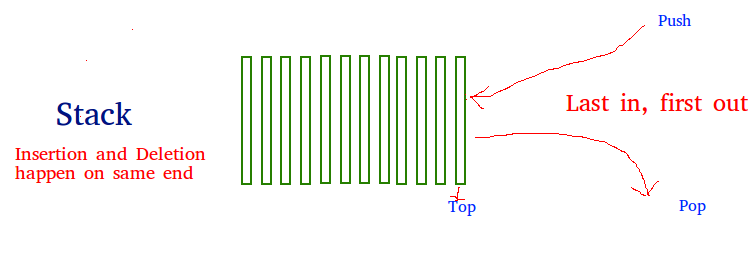The functions associated with stack are:

• empty() – Returns whether the stack is empty – Time Complexity: O(1)
• size() – Returns the size of the stack – Time Complexity: O(1)
• top() – Returns a reference to the topmost element of the stack – Time Complexity: O(1)
• push(a) – Inserts the element ‘a’ at the top of the stack – Time Complexity: O(1)
• pop() – Deletes the topmost element of the stack – Time Complexity: O(1)

### Python Stack Implementation

Stack in Python can be implemented using the following ways:

• list
• Collections.deque
• queue.LifoQueue

Implementation using List

Python’s built-in data structure list can be used as a stack. Instead of push(), append() is used to add elements to the top of the stack while pop() removes the element in LIFO order.

## Python3

 `stack ``=` `[]` `# append() function to push``# element in the stack``stack.append(``'g'``)``stack.append(``'f'``)``stack.append(``'g'``)` `print``(``'Initial stack'``)``print``(stack)` `# pop() function to pop``# element from stack in``# LIFO order``print``(``'\nElements popped from stack:'``)``print``(stack.pop())``print``(stack.pop())``print``(stack.pop())` `print``(``'\nStack after elements are popped:'``)``print``(stack)` `# uncommenting print(stack.pop())``# will cause an IndexError``# as the stack is now empty`
Output
```Initial stack
['g', 'f', 'g']

Elements popped from stack:
g
f
g

Stack after elements are popped:
[]```

Implementation using collections.deque:

Python stack can be implemented using the deque class from the collections module. Deque is preferred over the list in the cases where we need quicker append and pop operations from both the ends of the container, as deque provides an O(1) time complexity for append and pop operations as compared to list which provides O(n) time complexity.

## Python3

 `from` `collections ``import` `deque` `stack ``=` `deque()` `# append() function to push``# element in the stack``stack.append(``'g'``)``stack.append(``'f'``)``stack.append(``'g'``)` `print``(``'Initial stack:'``)``print``(stack)` `# pop() function to pop``# element from stack in``# LIFO order``print``(``'\nElements popped from stack:'``)``print``(stack.pop())``print``(stack.pop())``print``(stack.pop())` `print``(``'\nStack after elements are popped:'``)``print``(stack)` `# uncommenting print(stack.pop())``# will cause an IndexError``# as the stack is now empty`
Output
```Initial stack:
deque(['g', 'f', 'g'])

Elements popped from stack:
g
f
g

Stack after elements are popped:
deque([])```

Implementation using queue module

The queue module also has a LIFO Queue, which is basically a Stack. Data is inserted into Queue using the put() function and get() takes data out from the Queue.

## Python3

 `from` `queue ``import` `LifoQueue` `# Initializing a stack``stack ``=` `LifoQueue(maxsize ``=` `3``)` `# qsize() show the number of elements``# in the stack``print``(stack.qsize())` `# put() function to push``# element in the stack``stack.put(``'g'``)``stack.put(``'f'``)``stack.put(``'g'``)` `print``(``"Full: "``, stack.full())``print``(``"Size: "``, stack.qsize())` `# get() function to pop``# element from stack in``# LIFO order``print``(``'\nElements popped from the stack'``)``print``(stack.get())``print``(stack.get())``print``(stack.get())` `print``(``"\nEmpty: "``, stack.empty())`
Output
```0
Full:  True
Size:  3

Elements popped from the stack
g
f
g

Empty:  True```

## Queue

As a stack, the queue is a linear data structure that stores items in a First In First Out (FIFO) manner. With a queue, the least recently added item is removed first. A good example of the queue is any queue of consumers for a resource where the consumer that came first is served first.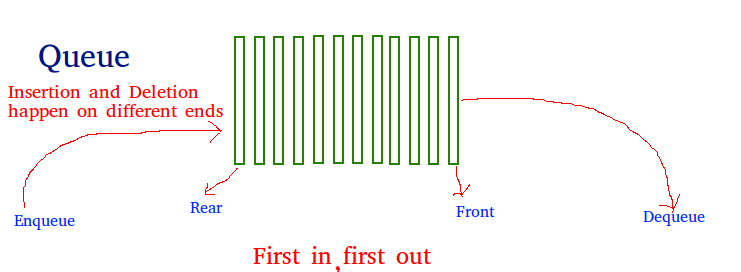Operations associated with queue are:

• Enqueue: Adds an item to the queue. If the queue is full, then it is said to be an Overflow condition – Time Complexity: O(1)
• Dequeue: Removes an item from the queue. The items are popped in the same order in which they are pushed. If the queue is empty, then it is said to be an Underflow condition – Time Complexity: O(1)
• Front: Get the front item from queue – Time Complexity: O(1)
• Rear: Get the last item from queue – Time Complexity: O(1)

### Python queue Implementation

Queue in Python can be implemented in the following ways:

• list
• collections.deque
• queue.Queue

Implementation using list

Instead of enqueue() and dequeue(), append() and pop() function is used.

## Python3

 `# Initializing a queue``queue ``=` `[]` `# Adding elements to the queue``queue.append(``'g'``)``queue.append(``'f'``)``queue.append(``'g'``)` `print``(``"Initial queue"``)``print``(queue)` `# Removing elements from the queue``print``(``"\nElements dequeued from queue"``)``print``(queue.pop(``0``))``print``(queue.pop(``0``))``print``(queue.pop(``0``))` `print``(``"\nQueue after removing elements"``)``print``(queue)` `# Uncommenting print(queue.pop(0))``# will raise and IndexError``# as the queue is now empty`
Output
```Initial queue
['g', 'f', 'g']

Elements dequeued from queue
g
f
g

Queue after removing elements
[]```

Implementation using collections.deque

Deque is preferred over the list in the cases where we need quicker append and pop operations from both the ends of the container, as deque provides an O(1) time complexity for append and pop operations as compared to list which provides O(n) time complexity.

## Python3

 `from` `collections ``import` `deque` `# Initializing a queue``q ``=` `deque()` `# Adding elements to a queue``q.append(``'g'``)``q.append(``'f'``)``q.append(``'g'``)` `print``(``"Initial queue"``)``print``(q)` `# Removing elements from a queue``print``(``"\nElements dequeued from the queue"``)``print``(q.popleft())``print``(q.popleft())``print``(q.popleft())` `print``(``"\nQueue after removing elements"``)``print``(q)` `# Uncommenting q.popleft()``# will raise an IndexError``# as queue is now empty`
Output
```Initial queue
deque(['g', 'f', 'g'])

Elements dequeued from the queue
g
f
g

Queue after removing elements
deque([])```

Implementation using the queue.Queue

queue.Queue(maxsize) initializes a variable to a maximum size of maxsize. A maxsize of zero ‘0’ means an infinite queue. This Queue follows the FIFO rule.

## Python3

 `from` `queue ``import` `Queue` `# Initializing a queue``q ``=` `Queue(maxsize ``=` `3``)` `# qsize() give the maxsize``# of the Queue``print``(q.qsize())` `# Adding of element to queue``q.put(``'g'``)``q.put(``'f'``)``q.put(``'g'``)` `# Return Boolean for Full``# Queue``print``(``"\nFull: "``, q.full())` `# Removing element from queue``print``(``"\nElements dequeued from the queue"``)``print``(q.get())``print``(q.get())``print``(q.get())` `# Return Boolean for Empty``# Queue``print``(``"\nEmpty: "``, q.empty())` `q.put(``1``)``print``(``"\nEmpty: "``, q.empty())``print``(``"Full: "``, q.full())` `# This would result into Infinite``# Loop as the Queue is empty.``# print(q.get())`
Output
```0

Full:  True

Elements dequeued from the queue
g
f
g

Empty:  True

Empty:  False
Full:  False```

## Priority Queue

Priority Queues are abstract data structures where each data/value in the queue has a certain priority. For example, In airlines, baggage with the title “Business” or “First-class” arrives earlier than the rest. Priority Queue is an extension of the queue with the following properties.

• An element with high priority is dequeued before an element with low priority.
• If two elements have the same priority, they are served according to their order in the queue.

## Python3

 `# A simple implementation of Priority Queue``# using Queue.``class` `PriorityQueue(``object``):``    ``def` `__init__(``self``):``        ``self``.queue ``=` `[]` `    ``def` `__str__(``self``):``        ``return` `' '``.join([``str``(i) ``for` `i ``in` `self``.queue])` `    ``# for checking if the queue is empty``    ``def` `isEmpty(``self``):``        ``return` `len``(``self``.queue) ``=``=` `0` `    ``# for inserting an element in the queue``    ``def` `insert(``self``, data):``        ``self``.queue.append(data)` `    ``# for popping an element based on Priority``    ``def` `delete(``self``):``        ``try``:``            ``max` `=` `0``            ``for` `i ``in` `range``(``len``(``self``.queue)):``                ``if` `self``.queue[i] > ``self``.queue[``max``]:``                    ``max` `=` `i``            ``item ``=` `self``.queue[``max``]``            ``del` `self``.queue[``max``]``            ``return` `item``        ``except` `IndexError:``            ``print``()``            ``exit()` `if` `__name__ ``=``=` `'__main__'``:``    ``myQueue ``=` `PriorityQueue()``    ``myQueue.insert(``12``)``    ``myQueue.insert(``1``)``    ``myQueue.insert(``14``)``    ``myQueue.insert(``7``)``    ``print``(myQueue)           ``    ``while` `not` `myQueue.isEmpty():``        ``print``(myQueue.delete())`
Output
```12 1 14 7
14
12
7
1```

## Heap queue (or heapq)

heapq module in Python provides the heap data structure that is mainly used to represent a priority queue. The property of this data structure in Python is that each time the smallest heap element is popped(min-heap). Whenever elements are pushed or popped, heap structure is maintained. The heap element also returns the smallest element each time.

It supports the extraction and insertion of the smallest element in the O(log n) times.

## Python3

 `# importing "heapq" to implement heap queue``import` `heapq` `# initializing list``li ``=` `[``5``, ``7``, ``9``, ``1``, ``3``]` `# using heapify to convert list into heap``heapq.heapify(li)` `# printing created heap``print` `(``"The created heap is : "``,end``=``"")``print` `(``list``(li))` `# using heappush() to push elements into heap``# pushes 4``heapq.heappush(li,``4``)` `# printing modified heap``print` `(``"The modified heap after push is : "``,end``=``"")``print` `(``list``(li))` `# using heappop() to pop smallest element``print` `(``"The popped and smallest element is : "``,end``=``"")``print` `(heapq.heappop(li))`
Output
```The created heap is : [1, 3, 9, 7, 5]
The modified heap after push is : [1, 3, 4, 7, 5, 9]
The popped and smallest element is : 1```

## Binary Tree

A tree is a  hierarchical data structure that looks like the below figure –

```      tree
----
j    <-- root
/   \
f      k
/   \      \
a     h      z    <-- leaves```

The topmost node of the tree is called the root whereas the bottommost nodes or the nodes with no children are called the leaf nodes. The nodes that are directly under a node are called its children and the nodes that are directly above something are called its parent.

A binary tree is a tree whose elements can have almost two children. Since each element in a binary tree can have only 2 children, we typically name them the left and right children. A Binary Tree node contains the following parts.

• Data
• Pointer to left child
• Pointer to the right child

## Python3

 `# A Python class that represents an individual node``# in a Binary Tree``class` `Node:``    ``def` `__init__(``self``,key):``        ``self``.left ``=` `None``        ``self``.right ``=` `None``        ``self``.val ``=` `key`

Now let’s create a tree with 4 nodes in Python. Let’s assume the tree structure looks like below –

```      tree
----
1    <-- root
/   \
2     3
/
4```

## Python3

 `# Python program to introduce Binary Tree` `# A class that represents an individual node in a``# Binary Tree``class` `Node:``    ``def` `__init__(``self``,key):``        ``self``.left ``=` `None``        ``self``.right ``=` `None``        ``self``.val ``=` `key`  `# create root``root ``=` `Node(``1``)``''' following is the tree after above statement``        ``1``    ``/ \``    ``None None'''` `root.left     ``=` `Node(``2``);``root.right     ``=` `Node(``3``);` `''' 2 and 3 become left and right children of 1``        ``1``        ``/ \``        ``2     3``    ``/ \ / \``None None None None'''`  `root.left.left ``=` `Node(``4``);``'''4 becomes left child of 2``        ``1``    ``/     \``    ``2         3``    ``/ \     / \``4 None None None``/ \``None None'''`

### Tree Traversal

Trees can be traversed in different ways. Following are the generally used ways for traversing trees. Let us consider the below tree –

```      tree
----
1    <-- root
/   \
2     3
/ \
4   5```

Depth First Traversals:

• Inorder (Left, Root, Right) : 4 2 5 1 3
• Preorder (Root, Left, Right) : 1 2 4 5 3
• Postorder (Left, Right, Root) : 4 5 2 3 1

Algorithm Inorder(tree)

• Traverse the left subtree, i.e., call Inorder(left-subtree)
• Visit the root.
• Traverse the right subtree, i.e., call Inorder(right-subtree)

Algorithm Preorder(tree)

• Visit the root.
• Traverse the left subtree, i.e., call Preorder(left-subtree)
• Traverse the right subtree, i.e., call Preorder(right-subtree)

Algorithm Postorder(tree)

• Traverse the left subtree, i.e., call Postorder(left-subtree)
• Traverse the right subtree, i.e., call Postorder(right-subtree)
• Visit the root.

## Python3

 `# Python program to for tree traversals` `# A class that represents an individual node in a``# Binary Tree``class` `Node:``    ``def` `__init__(``self``, key):``        ``self``.left ``=` `None``        ``self``.right ``=` `None``        ``self``.val ``=` `key`  `# A function to do inorder tree traversal``def` `printInorder(root):` `    ``if` `root:` `        ``# First recur on left child``        ``printInorder(root.left)` `        ``# then print the data of node``        ``print``(root.val),` `        ``# now recur on right child``        ``printInorder(root.right)`  `# A function to do postorder tree traversal``def` `printPostorder(root):` `    ``if` `root:` `        ``# First recur on left child``        ``printPostorder(root.left)` `        ``# the recur on right child``        ``printPostorder(root.right)` `        ``# now print the data of node``        ``print``(root.val),`  `# A function to do preorder tree traversal``def` `printPreorder(root):` `    ``if` `root:` `        ``# First print the data of node``        ``print``(root.val),` `        ``# Then recur on left child``        ``printPreorder(root.left)` `        ``# Finally recur on right child``        ``printPreorder(root.right)`  `# Driver code``root ``=` `Node(``1``)``root.left ``=` `Node(``2``)``root.right ``=` `Node(``3``)``root.left.left ``=` `Node(``4``)``root.left.right ``=` `Node(``5``)``print``(``"Preorder traversal of binary tree is"``)``printPreorder(root)` `print``(``"\nInorder traversal of binary tree is"``)``printInorder(root)` `print``(``"\nPostorder traversal of binary tree is"``)``printPostorder(root)`
Output
```Preorder traversal of binary tree is
1
2
4
5
3

Inorder traversal of binary tree is
4
2
5
1
3

Postorder traversal of binary tree is
4
5
2
3
1```

Time Complexity –  O(n)

Level order traversal of a tree is breadth-first traversal for the tree. The level order traversal of the above tree is 1 2 3 4 5.

For each node, first, the node is visited and then its child nodes are put in a FIFO queue. Below is the algorithm for the same –

• Create an empty queue q
• temp_node = root /*start from root*/
• Loop while temp_node is not NULL
• print temp_node->data.
• Enqueue temp_node’s children (first left then right children) to q
• Dequeue a node from q

## Python3

 `# Python program to print level``# order traversal using Queue` `# A node structure``class` `Node:``  ` `    ``# A utility function to create a new node``    ``def` `__init__(``self` `,key):``        ``self``.data ``=` `key``        ``self``.left ``=` `None``        ``self``.right ``=` `None` `# Iterative Method to print the``# height of a binary tree``def` `printLevelOrder(root):``  ` `    ``# Base Case``    ``if` `root ``is` `None``:``        ``return``    ` `    ``# Create an empty queue``    ``# for level order traversal``    ``queue ``=` `[]` `    ``# Enqueue Root and initialize height``    ``queue.append(root)` `    ``while``(``len``(queue) > ``0``):``    ` `        ``# Print front of queue and``        ``# remove it from queue``        ``print` `(queue[``0``].data)``        ``node ``=` `queue.pop(``0``)` `        ``# Enqueue left child``        ``if` `node.left ``is` `not` `None``:``            ``queue.append(node.left)` `        ``# Enqueue right child``        ``if` `node.right ``is` `not` `None``:``            ``queue.append(node.right)` `# Driver Program to test above function``root ``=` `Node(``1``)``root.left ``=` `Node(``2``)``root.right ``=` `Node(``3``)``root.left.left ``=` `Node(``4``)``root.left.right ``=` `Node(``5``)` `print` `(``"Level Order Traversal of binary tree is -"``)``printLevelOrder(root)`
Output
```Level Order Traversal of binary tree is -
1
2
3
4
5```

Time Complexity: O(n)

## Graph

A graph is a nonlinear data structure consisting of nodes and edges. The nodes are sometimes also referred to as vertices and the edges are lines or arcs that connect any two nodes in the graph. More formally a Graph can be defined as a Graph consisting of a finite set of vertices(or nodes) and a set of edges that connect a pair of nodes.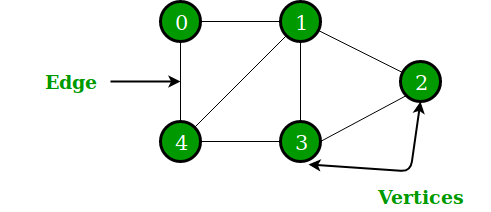In the above Graph, the set of vertices V = {0,1,2,3,4} and the set of edges E = {01, 12, 23, 34, 04, 14, 13}.

The following two are the most commonly used representations of a graph.

Adjacency Matrix is a 2D array of size V x V where V is the number of vertices in a graph. Let the 2D array be adj[][], a slot adj[i][j] = 1 indicates that there is an edge from vertex i to vertex j. The adjacency matrix for an undirected graph is always symmetric. Adjacency Matrix is also used to represent weighted graphs. If adj[i][j] = w, then there is an edge from vertex i to vertex j with weight w.

## Python3

 `# A simple representation of graph using Adjacency Matrix``class` `Graph:``    ``def` `__init__(``self``,numvertex):``        ``self``.adjMatrix ``=` `[[``-``1``]``*``numvertex ``for` `x ``in` `range``(numvertex)]``        ``self``.numvertex ``=` `numvertex``        ``self``.vertices ``=` `{}``        ``self``.verticeslist ``=``[``0``]``*``numvertex` `    ``def` `set_vertex(``self``,vtx,``id``):``        ``if` `0``<``=``vtx<``=``self``.numvertex:``            ``self``.vertices[``id``] ``=` `vtx``            ``self``.verticeslist[vtx] ``=` `id` `    ``def` `set_edge(``self``,frm,to,cost``=``0``):``        ``frm ``=` `self``.vertices[frm]``        ``to ``=` `self``.vertices[to]``        ``self``.adjMatrix[frm][to] ``=` `cost``        ` `        ``# for directed graph do not add this``        ``self``.adjMatrix[to][frm] ``=` `cost` `    ``def` `get_vertex(``self``):``        ``return` `self``.verticeslist` `    ``def` `get_edges(``self``):``        ``edges``=``[]``        ``for` `i ``in` `range` `(``self``.numvertex):``            ``for` `j ``in` `range` `(``self``.numvertex):``                ``if` `(``self``.adjMatrix[i][j]!``=``-``1``):``                    ``edges.append((``self``.verticeslist[i],``self``.verticeslist[j],``self``.adjMatrix[i][j]))``        ``return` `edges``        ` `    ``def` `get_matrix(``self``):``        ``return` `self``.adjMatrix` `G ``=``Graph(``6``)``G.set_vertex(``0``,``'a'``)``G.set_vertex(``1``,``'b'``)``G.set_vertex(``2``,``'c'``)``G.set_vertex(``3``,``'d'``)``G.set_vertex(``4``,``'e'``)``G.set_vertex(``5``,``'f'``)``G.set_edge(``'a'``,``'e'``,``10``)``G.set_edge(``'a'``,``'c'``,``20``)``G.set_edge(``'c'``,``'b'``,``30``)``G.set_edge(``'b'``,``'e'``,``40``)``G.set_edge(``'e'``,``'d'``,``50``)``G.set_edge(``'f'``,``'e'``,``60``)` `print``(``"Vertices of Graph"``)``print``(G.get_vertex())` `print``(``"Edges of Graph"``)``print``(G.get_edges())` `print``(``"Adjacency Matrix of Graph"``)``print``(G.get_matrix())`

Output

Vertices of Graph

[‘a’, ‘b’, ‘c’, ‘d’, ‘e’, ‘f’]

Edges of Graph

[(‘a’, ‘c’, 20), (‘a’, ‘e’, 10), (‘b’, ‘c’, 30), (‘b’, ‘e’, 40), (‘c’, ‘a’, 20), (‘c’, ‘b’, 30), (‘d’, ‘e’, 50), (‘e’, ‘a’, 10), (‘e’, ‘b’, 40), (‘e’, ‘d’, 50), (‘e’, ‘f’, 60), (‘f’, ‘e’, 60)]

[[-1, -1, 20, -1, 10, -1], [-1, -1, 30, -1, 40, -1], [20, 30, -1, -1, -1, -1], [-1, -1, -1, -1, 50, -1], [10, 40, -1, 50, -1, 60], [-1, -1, -1, -1, 60, -1]]

An array of lists is used. The size of the array is equal to the number of vertices. Let the array be an array[]. An entry array[i] represents the list of vertices adjacent to the ith vertex. This representation can also be used to represent a weighted graph. The weights of edges can be represented as lists of pairs. Following is the adjacency list representation of the above graph.## Python3

 `# A class to represent the adjacency list of the node``class` `AdjNode:``    ``def` `__init__(``self``, data):``        ``self``.vertex ``=` `data``        ``self``.``next` `=` `None`  `# A class to represent a graph. A graph``# is the list of the adjacency lists.``# Size of the array will be the no. of the``# vertices "V"``class` `Graph:``    ``def` `__init__(``self``, vertices):``        ``self``.V ``=` `vertices``        ``self``.graph ``=` `[``None``] ``*` `self``.V` `    ``# Function to add an edge in an undirected graph``    ``def` `add_edge(``self``, src, dest):``      ` `        ``# Adding the node to the source node``        ``node ``=` `AdjNode(dest)``        ``node.``next` `=` `self``.graph[src]``        ``self``.graph[src] ``=` `node` `        ``# Adding the source node to the destination as``        ``# it is the undirected graph``        ``node ``=` `AdjNode(src)``        ``node.``next` `=` `self``.graph[dest]``        ``self``.graph[dest] ``=` `node` `    ``# Function to print the graph``    ``def` `print_graph(``self``):``        ``for` `i ``in` `range``(``self``.V):``            ``print``(``"Adjacency list of vertex {}\n head"``.``format``(i), end``=``"")``            ``temp ``=` `self``.graph[i]``            ``while` `temp:``                ``print``(``" -> {}"``.``format``(temp.vertex), end``=``"")``                ``temp ``=` `temp.``next``            ``print``(``" \n"``)`  `# Driver program to the above graph class``if` `__name__ ``=``=` `"__main__"``:``    ``V ``=` `5``    ``graph ``=` `Graph(V)``    ``graph.add_edge(``0``, ``1``)``    ``graph.add_edge(``0``, ``4``)``    ``graph.add_edge(``1``, ``2``)``    ``graph.add_edge(``1``, ``3``)``    ``graph.add_edge(``1``, ``4``)``    ``graph.add_edge(``2``, ``3``)``    ``graph.add_edge(``3``, ``4``)` `    ``graph.print_graph()`
Output
```Adjacency list of vertex 0

head -> 4 -> 3 -> 2 -> 0

head -> 4 -> 2 -> 1

head -> 3 -> 1 -> 0 ```

### Graph Traversal

Breadth-First Traversal for a graph is similar to Breadth-First Traversal of a tree. The only catch here is, unlike trees, graphs may contain cycles, so we may come to the same node again. To avoid processing a node more than once, we use a boolean visited array. For simplicity, it is assumed that all vertices are reachable from the starting vertex.

For example, in the following graph, we start traversal from vertex 2. When we come to vertex 0, we look for all adjacent vertices of it. 2 is also an adjacent vertex of 0. If we don’t mark visited vertices, then 2 will be processed again and it will become a non-terminating process. A Breadth-First Traversal of the following graph is 2, 0, 3, 1.## Python3

 `# Python3 Program to print BFS traversal``# from a given source vertex. BFS(int s)``# traverses vertices reachable from s.``from` `collections ``import` `defaultdict` `# This class represents a directed graph``# using adjacency list representation``class` `Graph:` `    ``# Constructor``    ``def` `__init__(``self``):` `        ``# default dictionary to store graph``        ``self``.graph ``=` `defaultdict(``list``)` `    ``# function to add an edge to graph``    ``def` `addEdge(``self``,u,v):``        ``self``.graph[u].append(v)` `    ``# Function to print a BFS of graph``    ``def` `BFS(``self``, s):` `        ``# Mark all the vertices as not visited``        ``visited ``=` `[``False``] ``*` `(``max``(``self``.graph) ``+` `1``)` `        ``# Create a queue for BFS``        ``queue ``=` `[]` `        ``# Mark the source node as``        ``# visited and enqueue it``        ``queue.append(s)``        ``visited[s] ``=` `True` `        ``while` `queue:` `            ``# Dequeue a vertex from``            ``# queue and print it``            ``s ``=` `queue.pop(``0``)``            ``print` `(s, end ``=` `" "``)` `            ``# Get all adjacent vertices of the``            ``# dequeued vertex s. If a adjacent``            ``# has not been visited, then mark it``            ``# visited and enqueue it``            ``for` `i ``in` `self``.graph[s]:``                ``if` `visited[i] ``=``=` `False``:``                    ``queue.append(i)``                    ``visited[i] ``=` `True` `# Driver code` `# Create a graph given in``# the above diagram``g ``=` `Graph()``g.addEdge(``0``, ``1``)``g.addEdge(``0``, ``2``)``g.addEdge(``1``, ``2``)``g.addEdge(``2``, ``0``)``g.addEdge(``2``, ``3``)``g.addEdge(``3``, ``3``)` `print` `(``"Following is Breadth First Traversal"``                ``" (starting from vertex 2)"``)``g.BFS(``2``)` `# This code is contributed by Neelam Yadav`
Output
```Following is Breadth First Traversal (starting from vertex 2)
2 0 3 1 ```

Time Complexity: O(V+E) where V is the number of vertices in the graph and E is the number of edges in the graph.

Depth First Search or DFS

Depth First Traversal for a graph is similar to Depth First Traversal of a tree. The only catch here is, unlike trees, graphs may contain cycles, a node may be visited twice. To avoid processing a node more than once, use a boolean visited array.

Algorithm:

• Create a recursive function that takes the index of the node and a visited array.
• Mark the current node as visited and print the node.
• Traverse all the adjacent and unmarked nodes and call the recursive function with the index of the adjacent node.

## Python3

 `# Python3 program to print DFS traversal``# from a given graph``from` `collections ``import` `defaultdict` `# This class represents a directed graph using``# adjacency list representation`  `class` `Graph:` `    ``# Constructor``    ``def` `__init__(``self``):` `        ``# default dictionary to store graph``        ``self``.graph ``=` `defaultdict(``list``)` `    ``# function to add an edge to graph``    ``def` `addEdge(``self``, u, v):``        ``self``.graph[u].append(v)` `    ``# A function used by DFS``    ``def` `DFSUtil(``self``, v, visited):` `        ``# Mark the current node as visited``        ``# and print it``        ``visited.add(v)``        ``print``(v, end``=``' '``)` `        ``# Recur for all the vertices``        ``# adjacent to this vertex``        ``for` `neighbour ``in` `self``.graph[v]:``            ``if` `neighbour ``not` `in` `visited:``                ``self``.DFSUtil(neighbour, visited)` `    ``# The function to do DFS traversal. It uses``    ``# recursive DFSUtil()``    ``def` `DFS(``self``, v):` `        ``# Create a set to store visited vertices``        ``visited ``=` `set``()` `        ``# Call the recursive helper function``        ``# to print DFS traversal``        ``self``.DFSUtil(v, visited)` `# Driver code`  `# Create a graph given``# in the above diagram``g ``=` `Graph()``g.addEdge(``0``, ``1``)``g.addEdge(``0``, ``2``)``g.addEdge(``1``, ``2``)``g.addEdge(``2``, ``0``)``g.addEdge(``2``, ``3``)``g.addEdge(``3``, ``3``)` `print``(``"Following is DFS from (starting from vertex 2)"``)``g.DFS(``2``)`
Output
```Following is DFS from (starting from vertex 2)
2 0 1 3 ```

Time complexity: O(V + E), where V is the number of vertices and E is the number of edges in the graph.

My Personal Notes arrow_drop_up